# Measurement of electrostatic tip–sample interactions by time-domain Kelvin probe force microscopy

1Nanotechnology Group, ETH Zürich, Säumerstrasse 4, 8803 Rüschlikon, Switzerland
2present address: Zurich Instruments AG, Technoparkstrasse 1, 8005 Zürich, Switzerland

1. Corresponding author email

Associate Editor: T. Glatzel
Beilstein J. Nanotechnol. 2020, 11, 911–921. https://doi.org/10.3762/bjnano.11.76
Received 26 Jan 2020, Accepted 05 May 2020, Published 15 Jun 2020

• Full Research Paper

## Abstract

Kelvin probe force microscopy is a scanning probe technique used to quantify the local electrostatic potential of a surface. In common implementations, the bias voltage between the tip and the sample is modulated. The resulting electrostatic force or force gradient is detected via lock-in techniques and canceled by adjusting the dc component of the tip–sample bias. This allows for an electrostatic characterization and simultaneously minimizes the electrostatic influence onto the topography measurement. However, a static contribution due to the bias modulation itself remains uncompensated, which can induce topographic height errors. Here, we demonstrate an alternative approach to find the surface potential without lock-in detection. Our method operates directly on the frequency-shift signal measured in frequency-modulated atomic force microscopy and continuously estimates the electrostatic influence due to the applied voltage modulation. This results in a continuous measurement of the local surface potential, the capacitance gradient, and the frequency shift induced by surface topography. In contrast to conventional techniques, the detection of the topography-induced frequency shift enables the compensation of all electrostatic influences, including the component arising from the bias modulation. This constitutes an important improvement over conventional techniques and paves the way for more reliable and accurate measurements of electrostatics and topography.

Keywords: atomic force microscopy (AFM); electrostatic height error; extended Kalman filter; Kelvin probe force microscopy (KFM); time domain

## Introduction

Electrostatic forces are important interactions in non-contact atomic force microscopy (NC-AFM). They arise from differences in the work function of the tip and the sample, from trapped charges, or from potentials applied to active nanoelectronic devices. Kelvin probe force microscopy (KFM) is a technique used to quantitatively characterize such electrical properties [1-3]. It is applied to map material compositions via changes in the work function, to localize charge distributions in dielectric samples [4,5], and to characterize doping profiles via scanning capacitance measurements . Especially in the field of nanoelectronic devices, this kind of electrical characterizations is of great interest. Local potential drops across active nanostructures reveal information about the local resistivity and can provide crucial insights into the operation mode [7,8].

The principle of KFM is based on modulating the electrostatic force or force gradient. To this end, the tip–sample bias voltage is set to

When modeling the tip–sample system as a capacitance, the resulting electrostatic force is defined as

where C is the tip–sample capacitance and Ulcpd is the local contact potential difference. Ulcpd contains information about both the contact potential difference and the potential arising from charge interactions [9,10]. Consequently, the electrostatic force gradient is given by

It should be noted that capacitance C can be a function of not only the distance z but also of the applied bias voltage Uts. This should be observed especially at large bias in conjunction with a semiconducting tip or sample due to band bending . In this case, the model can be extended by considering the Taylor expansion of the capacitance with respect to the tip–sample bias, defined by

Different spectral components arise due to the electric modulation at ωm. Namely, a static component (dc) as well as components at the modulation frequency ωm and at the second harmonic frequency 2ωm. These spectral components are defined by

with

and

In conventional frequency-modulated (FM-) KFM, the contributions at ωm and 2ωm are detected via lock-in techniques, either at the Δf output of a phase-locked loop (PLL)  or by detecting the sidebands of the cantilever oscillation .

In closed-loop FM-KFM, a feedback loop is employed to nullify the component at ωm by adjusting Udc. The surface potential is then found as Ulcpd = Udc. The response at the second harmonic contains additional information about the capacitance gradient C′′ = ∂2C/∂z2. This signal is interesting in itself as it contains information about both geometric and electronic properties of tip and sample, e.g., the dielectric properties of a sample or the quantum capacitance . Furthermore, this signal can be used to adjust the sensitivity of the KFM feedback loop .

Open-loop KFM techniques exploit the relationship of the contributions at ωm and 2ωm. Namely, Ulcpd can be found from their ratiowhich otherwise only depends on the parameters of the applied modulation, Uac and Udc. When applying KFM as an open-loop technique, optimization of filter parameters is possible, leading to an improved controller performance .

For correct height measurements, it is necessary to compensate the electrostatic forces [17-19]. With a closed KFM loop, these forces are minimized by definition. However, a static contribution, which is defined by

remains uncompensated. This component results from the bias modulation itself and influences the topography measurement. The effect is amplified when a significant change in the capacitance gradient is present. To reduce the impact of this component onto the height measurement, the modulation amplitude Uac must be minimized. Since the signal-to-noise ratio (SNR) scales with Uac, this is only possible to a certain extent. Another possibility for compensating the remaining frequency shift is the use of two-pass methods with feed-forward compensation techniques [20,21].

In this paper, we present a time-domain (TD) controller for KFM as a single-pass solution to the problem outlined above. Our method uses a Kalman filter as a state observer to continuously recover the full Δf(Uts) parabola, also named Kelvin parabola. The maximum frequency shift Δftopo, the contact potential difference Ulcpd, and the capacitance gradient C′′ are evaluated in real time.

When applied as closed-loop technique, the height feedback can be performed on Δftopo, where all electrostatic forces are compensated, including the static contribution. So far, recovering and fitting the Kelvin parabola is known as an open-loop technique, the so-called Kelvin probe force spectroscopy [22-25]. A real-time closed-loop technique has not been reported yet.

Furthermore, our method automatically determines the estimated error signals and the signal correlation coefficients. The error signals of the sample properties contain an evaluation of the accuracy at each position, which depends on the present surface properties due to the nonlinearity of the system. The signal correlation coefficients contain information about coupling and crosstalk between the channels estimated. Our method can be applied in closed-loop as well as in open-loop mode. Results of both modes are discussed in this paper.

## Detection Principle

The overall frequency shift in FM-AFM can be separated into a component induced by surface topography, Δftopo, and a component induced electrically, Δfel, therefore

The coefficient a is proportional to the capacitance gradient C′′ and has the unit of Hz V−2. It is one of the three sample properties that are continuously estimated by the controller. The additional two estimated properties are Δftopo and the surface potential Ulcpd.

By applying a sinusoidal modulation to the tip–sample bias Uts, the parabolic dependence in Equation 10 can be observed. An example of the resulting Kelvin parabola is shown in Figure 1. The data was obtained above a single-layer graphene flake. The figure shows the influence of the three sample properties on the shape of the parabola and gives an overview of the different components of the frequency shift Δf.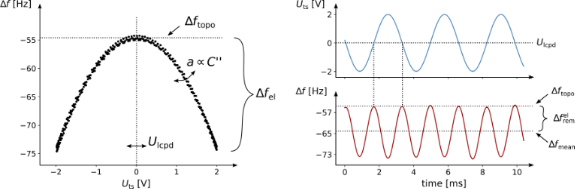Figure 1: A modulation of the tip–sample bias Uts leads to a response in the frequency shift Δf. The time-resolved measurements of both signals are shown on the right, the resulting Kelvin parabola on the left. From this information, the controller continuously estimates the contact potential difference Ulcpd, the capacitance gradient C′′, and the frequency shift due to the topography alone, Δftopo. Sample: Single-layer graphene on SiO2; Tip: Olympus AC240TM-R3. Figure 1: A modulation of the tip–sample bias Uts leads to a response in the frequency shift Δf. The time-res... Jump to Figure 1

Traditional closed-loop controllers for KFM use lock-in techniques to measure the response of the cantilever at the modulation frequency ωm (not visible in Figure 1 since UdcUlcpd) and at 2ωm [13,26,27]. A feedback loop is used to adjust Udc in order to nullify the component at ωm. If this is achieved, the resulting Δfmean, which is used for the topography control, is independent of the surface potential. However, it is still affected by the static contribution Δfrem. As can be seen in Equation 9, this component depends on C′′ and may change across material borders, inducing a topographic height error.

The approach discussed in this paper tracks the time-resolved Δf-response to the applied bias voltage. A state observer based on an extended Kalman filter is used to continuously fit the resulting parabola. The output of the time-based controller is an estimation of the topography-induced frequency shift Δftopo (which is not affected by Δfrem), the surface potential Ulcpd, and the coefficient a. Since our technique is based on the time domain, it is not limited by the bandwidth of additional filters in the loop, for example lock-in amplifiers. Therefore, we expect to achieve higher bandwidths compared to standard KFM implementations.

An asymmetrical bias dependence, as caused by band bending, may be included. When considering the Taylor expansion in Equation 4, the overall frequency shift results in

The sample property b is introduced to the estimator as an additional state. This gives the controller the ability to locally fit a third-order parabola to the KFM data. Similarly, other sources of asymmetry may be included here, although the detectability of the system needs to be confirmed. For instance, the polarizability of a sample could be added through an additional state γ and by modifying Equation 10, e.g., according to Equation 8 in .

For the sake of simplicity, this publication focusses on the implementation of the most basic case with three states, where only the quadratic influence on the Kelvin parabola is considered. The model of the measurement system, which is used by the state observer, has to include the dynamics of the detection system. If a PLL is used, its transfer function, which is known for a given cantilever and given PI gains, can be approximated by the transfer function of a low-pass filter.

Using the relationship shown in Equation 10 and the transfer function of the detection system, the sample properties, the expected error signals, and the correlation coefficients can be determined from the bias dependence of the frequency shift Δf(Uts). For robust estimation, it is beneficial if the surface potential Ulcpd is within the modulated bias voltage Uts(t). A single-sided modulation on one branch is feasible but not recommended.

## Controller Design

The state-space representation of the Kelvin system and the detection system are derived separately, using the superscripts K and D, respectively. The two subsystems will be merged in Equations 22–26. An illustration of the two subsystems in the overall KFM model is shown in Figure 2a.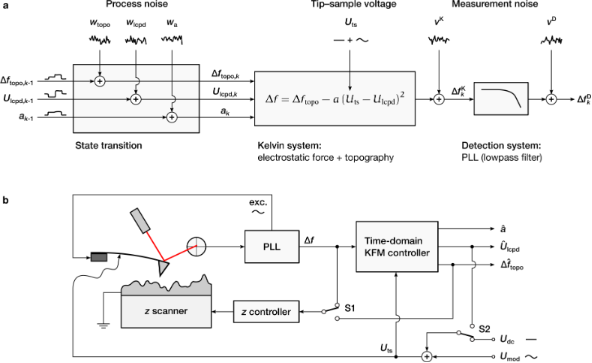Figure 2: Design and operation of the time-domain KFM controller. (a) Underlying system model. (b) Measurement setup. The estimated topography-induced frequency shift, , can be used in place of Δf to minimize electrostatic height errors (switch S1). The electrostatic force gradient between tip and sample is minimized by adding as a dc bias to Uts (switch S2). Figure 2: Design and operation of the time-domain KFM controller. (a) Underlying system model. (b) Measuremen... Jump to Figure 2

The Kelvin system, i.e., the measurement system without the detector, has the state vector

Index k is the discrete sampling applied, tk = kΔt. The model assumes that the sample properties remain constant between two discrete measurements, thus

Deviations from this assumption, for example due to scan movement, are introduced by the vector

This vector, the so-called transition noise vector, is anticipated to be Gaussian white noise(0,QK) with the covariance matrix

The output of the Kelvin system is the scalar value of the intrinsic frequency shift. It is defined by

using the definition in Equation 10. The measurement noiseis assumed to be Gaussian white noise and is defined by. This is the case for measurements where thermal noise is the dominating noise source, i.e., when the modulation frequency is below the crossover of thermal white noise and (with increasing frequency) detection noise . The power spectral density (PSD) of the thermal noise can be calculated as

For detection-noise-limited measurements, an additional state has to be introduced to keep track of the dynamics of the apparent noise.

The approximation in Equation 17 contains the system output matrix. It depends on the current tip–sample voltage Uts,k and linearizes the system around the state. It is defined by

The intrinsic frequency shift,, then enters the detection system, which is modeled by the linear time-invariant system represented by the state transition matrix FD, input matrix GD, output matrix HD, and feed-through coefficient DD:

The observed frequency shift Δfk corresponds to the output of the detection system,. If a PLL is used as a detection technique, the above system can be approximated by a low-pass filter. The noise introduced at the output of the detection system is modeled by Gaussian white noise with.

Merging the two subsystems leads to the state-space representation given by

with

and the transition and observation noise

The state observer uses the derived state-space description of the measurement system for continuously evaluating xK, i.e., the sample properties, according to the measured frequency shift. Due to the nonlinearity of the system, nonlinear state estimation is required. The extended Kalman filter (EKF) is a straightforward and computationally efficient method for dealing with such systems. The implementation shown uses the notation of Simon .

The algorithm starts with an arbitrary initialization of the a posteriori state estimateand the initial state covariance matrix(e.g.,and). Afterwards, two steps have to be applied for each iteration k. The prediction step calculates the a priori estimatefrom the previous a posteriori estimateaccording to

Note that Fk−1 contains the linearization through the output matrixand has to be updated beforehand. The linearization step shown in Equation 19 is performed around the last estimateand the known bias voltage Uts,k−1. Similarly, the system a priori covariance matrixis calculated fromas in

with the transition noise matrix

The transition noise matrix of the detection system QD is the zero matrix with the PSD of the thermal noise Rth at its top-left position. The covariance matrix QK contains the assumed PSD of the sample properties. The values of QK are design parameters and determine how much change may be expected between two sampled points. For the TD controller, these values represent the gain of each estimated channel. The sampling-rate-independent continuous noise density QK (QD) is divided (multiplied) by fs to obtain the discrete noise density Q.

After the prediction step, a measurement update is performed. The Kalman gain Kk is calculated as

It determines how much weight should be put onto the currently measured Δfk. Again, Hk has to be updated before using Equation 19 where the linearization is made around the time-updated stateand the current bias voltage Uts,k.

The a posteriori estimateand covarianceare then calculated as

The a posteriori values are considered to be the best estimates at time k. During the estimation, a negative value for the coefficient a may result. Due to the definition in Equation 10, this is physically not valid and can be corrected by projecting stateback to the allowed region .

The state covariance matrixprovides additional information about the measurement. The estimated variances σi,k of state xk are the diagonal values ofand represent the expected error signals. These values have to be interpreted carefully since they depend on the design parameters in QK. A small value for Qi is interpreted as an increased confidence towards the prediction of this channel resulting in a smaller variance. However, due to the nonlinearity of the measurement system, the error signals also depend on the estimated sample properties. This allows one to distinguish regions where an increased SNR can be expected from regions where the controller is less confident due to a smaller SNR.

Furthermore, the correlation coefficients can be calculated as

They contain information about coupling and crosstalk between the different states. If the magnitude of a correlation coefficient is close to one, crosstalk between these two channels has to be expected, while a value close to zero means little to no crosstalk.

The measurement setup is shown in Figure 2b. The estimator can be applied in both open-loop and closed-loop mode. When usingas control signal for the topography measurement (switch S1), the controller aims to nullify the electrostatic height error. The electrostatic force between tip and sample is minimized when addingas dc bias to Uts (switch S2). If the topography control usesas feedback signal, the electrostatic forces are compensated already, even for an open KFM loop. Nevertheless, closing the KFM loop prevents a single-sided modulation where the controller is much less sensitive and may lose its stability.

To initially tune the closed-loop controller, it helped to approach the sample with Δf as feedback parameter and an open KFM loop (open S1 and S2). After approaching the surface, the input signals Δf and Uts of the estimator and the reconstructed surface propertiesandwere observed on an external oscilloscope. The controller could be stabilized by adjusting the values of the transition noise matrix QK. After the output signals of the estimator were steady, both switches were closed. Note that the topography setpoint should be adjusted before closing S1 because Δftopo ≥ Δfmean as illustrated in Figure 1.

## Results and Discussion

The performance of the TD-KFM controller is demonstrated on multilayer graphene samples. The samples were obtained by exfoliation from bulk graphite [32,33] and deposited on a piece of Si/SiO2. Measurements were carried out using an Asylum Research Cypher AFM connected to a Zurich Instruments HF2 lock-in amplifier.

Figure 3 shows the results of the open-loop controller. During the FM-AFM measurement the tip voltage was modulated with an amplitude of Uac = 1 V at fm = 500 Hz. The frequency shift Δf was obtained by using a PLL with a bandwidth set to 1.5 kHz, such that the electrostatic contributions at fm and 2fm were detected. Both tip voltage and frequency shift were recorded at a sampling rate of fs = 3.6 kHz.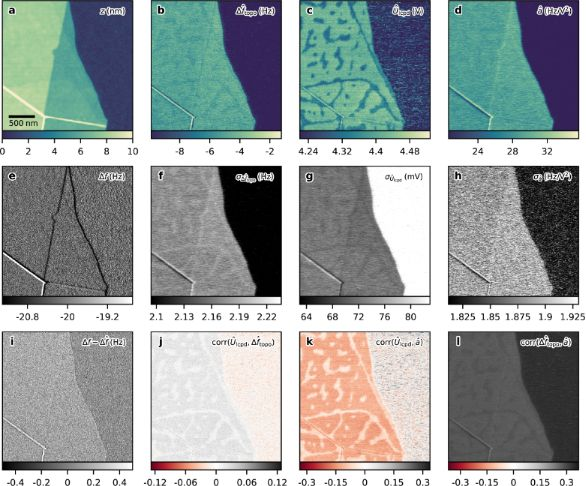Figure 3: Open-loop TD-KFM of a few-layer graphene flake. (a) Topography, (b) estimated topography-induced frequency shift , (c) estimated surface potential , and (d) estimated coefficient . (e) The frequency shift Δf was kept constant at −20 Hz by the topography feedback. (f–h) and (j–l) show the variance and mutual correlations of the estimated states, respectively. (i) Residual of the estimation. Parameters: Uac = 1 V, Udc = 4.3 V, fm = 500 Hz, fs = 3.6 kHz, PLL bandwidth: 1.5 kHz, Rth = 1.55 × 10−3 Hz2 Hz−1, Qtopo = 4800 Hz2 Hz, Qlcpd = 10 V2 Hz, Qa = 400 Hz2 V−4 Hz; Detection noise RD ≪ Rth is neglected here. Tip: Olympus AC240TM-R3, A = 14 nm, k = 2.3 N m−1, f0 = 71.04 kHz, Q = 134, vscan = 2.5 μm s−1. Figure 3: Open-loop TD-KFM of a few-layer graphene flake. (a) Topography, (b) estimated topography-induced fr... Jump to Figure 3

The surface topography is shown in Figure 3a, the obtained frequency shift is shown in Figure 3e. During postprocessing, the recorded Δf signal and bias voltage are fed to the TD-KFM controller. From the hidden contributions at fm and 2fm, the state observer reconstructed the sample properties, which are shown in Figure 3b–d. The topography-induced frequency shift Δftopo in Figure 3b is far from the −20 Hz Δf setpoint used for the height feedback, indicating a large influence of the electrostatic force gradient. The material contrast present can be explained partially by differences in the surface potential, which was not compensated during this measurement, and partially by differing contributions due to. This contribution causes the bias-induced height error and will be further discussed below for the closed-loop demonstration. The estimate of the surface potential, depicted in Figure 3c, reveals the electrostatic potential above the graphene flake and the SiO2 substrate. The patterns observable on the graphene flake are most likely caused by water droplets, which have formed due to the ambient conditions [34,35]. Small changes in the patterns were observed between two different scans (roughly 20 minutes apart) due to adsorption and desorption. The estimate of coefficient a is depicted in Figure 3d, showing a material contrast caused by the difference in the capacitance gradient. The expected state variances are shown in Figure 3f–h. Due to the nonlinearity of the KFM system, the uncertainty of an estimated channel depends on the present state vector. A larger value for coefficient a, for example, increases the SNR of the surface potential measurement, leading to a smaller variance. A larger confidence is then attributed to the Ulcpd channel. Consequently, the expected error of the surface potential above the graphene flake is smaller than that above the SiO2 substrate. The residual of the state observeris shown in Figure 3i. This signal contains the amount of the frequency shift that could not be attributed to any surface property, for example the estimated measurement noise. In the present case, a material-dependent offset is visible. A mean value of 0.15 Hz can be extracted above the graphene flake while an average value of 0.07 Hz is found above the SiO2 substrate. This material-dependent component indicates the presence of coefficient b, which was approximated to be zero in the model assumptions. The presence and the magnitude of coefficient b are discussed in Supporting Information File 1, Figure S1. Figure 3j–l shows the correlation coefficients of the sample properties.

In the following, we demonstrate the application of the TD controller as a closed-loop technique. The sample examined consists of two overlapping single-layer graphene sheets, forming a bilayer in the lower part of the image. For comparing the TD controller to state-of-the-art KFM, the graphene flake was initially scanned by standard FM-KFM using sideband demodulation. A Kalman filter was used for optimal feedback control during this measurement . The results of the standard technique are shown in Figure 4a–c. A second measurement was carried out using the TD-KFM controller. The topography-induced frequency shiftwas used as control signal for the height feedback in this case. The results obtained from the state observer are depicted in Figure 4e–h.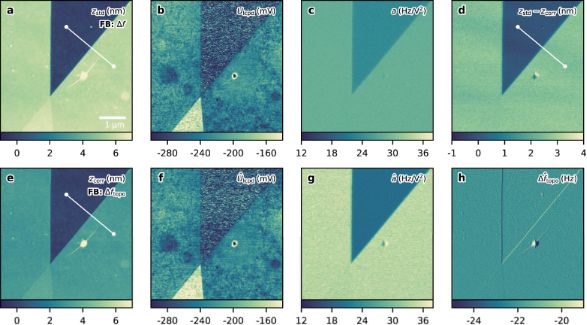Figure 4: Comparison of standard single-scan FM-KFM and closed-loop TD-KFM of two overlapping graphene flakes. (a–c) Topography, Ulcpd, and coefficient a obtained from a FM-KFM scan with direct sideband detection. (e–h) Topography, , coefficient , and obtained by TD-KFM. The estimated topography-induced frequency shift was used as topography feedback here. (d) Height difference between the TD-KFM and standard FM-KFM scan. The cross sections indicated by the white lines are shown below in Figure 5. Parameters FM-KFM: Δfset = −65 Hz, Uac = 2 V, fm = 1.5 kHz, PLL bandwidth: 500 Hz; TD-KFM: = −22 Hz, Uac = 2 V, fm = 253 Hz, fs = 3.6 kHz, PLL bandwidth: 1 kHz, Rth = 1.66 × 10−3 Hz2 Hz−1, Qtopo = 5 Hz2 Hz, Qlcpd = 0.004 V2 Hz, Qa = 5 Hz2 V−4 Hz; Detection noise RD ≪ Rth is neglected here. Tip: Olympus AC240TM-R3, f0 = 52.0 kHz, k = 1.0 N m−1, Q = 77.2, A = 12 nm (active ACL), vscan = 1 μm s−1. Figure 4: Comparison of standard single-scan FM-KFM and closed-loop TD-KFM of two overlapping graphene flakes... Jump to Figure 4

The topography measurement of the standard KFM technique is influenced by the static contribution. The contribution is proportional to the coefficient a and, thus, changes across the border of the graphene flake. The larger capacitance gradient present in the region of the graphene flake (see Figure 4c and Figure 4g) causes a more negative total frequency shift, which leads to an additional retraction of the tip. Consequently, an exaggerated height is measured. The TD controller is not affected by this artifact.

The difference between the two topography measurements is shown in Figure 4d. The height difference caused by the presence ofis roughly 2.6 nm, which is a large portion of the height measured by standard FM-KFM. This points out the importance of compensating the additional static contributioninduced by the electric modulation.

The height profiles shown in Figure 5 are extracted from the topography images across the border of the graphene flake, as is indicated in Figure 4. Figure 5a shows the measured topography for both techniques and Figure 5b shows the obtained difference.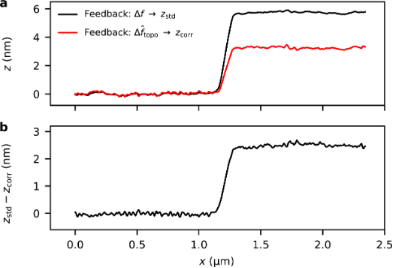Figure 5: (a) Cross sections of the topography along the sections indicated in Figure 4 for single-scan FM-KFM (feedback on Δf) and closed-loop TD-KFM (feedback on ). (b) Difference between both topography measurements. Closed-loop TD-KFM minimizes the electrostatic height error beyond what is achievable in standard FM-KFM. Figure 5: (a) Cross sections of the topography along the sections indicated in Figure 4 for single-scan FM-KFM (feedb... Jump to Figure 5

Standard FM-KFM and TD-KFM found similar values for the surface potential Ulcpd. In both cases, the resolution depends on the controller settings and the same physical limitations apply. The results of the surface potential measurements are shown in Figure 4b and Figure 4f. The potential difference between the single- and the bilayer graphene is roughly 70 mV, which is similar to previously reported values .

The measurements of coefficient a are slightly different for the two techniques. It has to be noted, that the capacitance gradient is largely influenced by the tip–sample distance. The average tip–sample separation during the measurement with the standard KFM technique was larger above the graphene flake and smaller above the SiO2 substrate when compared to the TD measurement. This led to an increased value for a above the SiO2 substrate and a decreased value above the graphene flake.

## Conclusion

We demonstrated that surface topography and electrostatic properties can be directly estimated and separated from the frequency-shift signal. The state-space representation of FM-KFM was derived and an extended Kalman filter was introduced as a state observer. The estimation uses a linearization around the output matrix of the Kelvin system.

In our method, the maximum frequency shift is detected in real time and can be used as control signal for the topography feedback. The maximum frequency shift, Δftopo, is not affected by the electrostatic force gradient, and therefore allows for the compensation of all electrostatic forces, including the remaining static contribution present in standard FM-KFM. The improved topography measurement was demonstrated by a closed-loop scan on a graphene flake.

The TD controller is also applicable as an open-loop technique. By modulating the tip–sample bias voltage during a regular FM-AFM measurement and by recording the frequency-shift signal and the applied bias voltage, the surface potential image can be reconstructed by the state observer. This is a very convenient way of performing KFM measurements by postprocessing. Furthermore, the dynamics of the controller can be studied and tested before conducting an actual closed-loop measurement.

The algorithm is reliable and robust for a wide range of gain values. The three parameters of the state transition matrix are the only tuning parameters needed, apart from the PSD of the thermal noise, which can be calculated beforehand.

## Code Availability

The code for the TD controller is made available upon request. It includes an open-loop version for postprocessing and a version for closed-loop application designed for the real-time unit (RTK) of the HF2 lock-in amplifier of Zurich Instruments.

## Supporting Information

 Supporting Information File 1: Evaluation of the third sideband of the measurement shown in Figure 3. Format: PDF Size: 1.4 MB Download

#### Interesting articles

##### Direct measurement of surface photovoltage by AC bias Kelvin probe force microscopy

Masato Miyazaki, Yasuhiro Sugawara and Yan Jun Li

##### Implementation of data-cube pump–probe KPFM on organic solar cells

Benjamin Grévin, Olivier Bardagot and Renaud Demadrille

##### Combined scanning probe electronic and thermal characterization of an indium arsenide nanowire

Tino Wagner, Fabian Menges, Heike Riel, Bernd Gotsmann and Andreas Stemmer

#### News

##### ROR allows to connect researchers and their outputs to affiliations##### Register for the Beilstein Nanotechnology Symposium 2022!##### We are looking for an active researcher who is interested in a unique opportunity with a non-profit diamond open access journal.This is an Open Access article under the terms of the Creative Commons Attribution License (http://creativecommons.org/licenses/by/4.0). Please note that the reuse, redistribution and reproduction in particular requires that the authors and source are credited.
The license is subject to the Beilstein Journal of Nanotechnology terms and conditions: (https://www.beilstein-journals.org/bjnano)